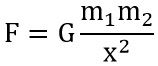# Difference Between Gravitation and Gravity

The terms gravitation and gravity both are associated with the force on a body present on the surface of the earth. The crucial difference between gravitation and gravity is that gravitation is associated with two objects present in the universe. While gravity is specifically applied between earth and a body present on its surface.

As the two terms are a bit confusing thus sometimes these are misinterpreted and people consider both as the same. However, various factors exist that differentiates gravitation from gravity.

## Comparison Chart

ParameterGravitationGravity
BasicIt is the force acting between two bodies in the universe.It is the force applied by earth on object present near it.
Type of forceAttractive or repulsiveAttractive only
Nature of forceWeak forceComparitively stronger than gravitational force
RequirementIt needs the mass of each individual body between which the force is to be determined.It needs the mass of single body present near the earth.
Given asValue of constantG is a universal constant,
G = 6.67 * 10-11 Nm2 / Kg2
g is not a universal constant,
g = 9.8 m/s2

### Definition of Gravitation

A natural phenomenon occurring between two distant objects placed in the universe, that causes them to move is known as gravitation.

We know that every body in this universe moves only under the influence of some force. So, the force acting between two bodies in the universe is known as the gravitational force. Let us consider the figure shown below:Here two bodies are separated at a distance x and the masses of the two objects be m1 and m2. So, the force acting between two bodies will be given as:Further: G specifies universal gravitational constant whose value is 6.67 * 10-11 NM2/Kg2.

As G is the universal constant hence its value is the same all over the universe. The force experienced by the bodies relies on their masses as well as the distance of their separation. So, the force between the bodies will be higher when the bodies are of large masses. However, the force will be very low in case the two are separated at a very large distance with respect to each other.

### Definition of Gravity

Gravity is also a natural phenomenon that acts between the earth and the object placed in the universe. The force of gravity is generally defined as the force acting between earth and an object present near it. Gravity is specifically associated with earth and the object present near it.

We know that earth is a body with extremely huge mass, and it is to be noted that the body of higher mass attracts the body having lower mass. Thus it is said that the force of gravity is attractive in nature, as earth attracts the body present near it.

Force of gravity is given as:: m specifies the body mass and

G is the acceleration due to gravity and its value is 9.8m/s2. However, this acceleration due to gravity is not a universal constant hence its value shows variation.

It is noteworthy that the gravitational force is extremely small in comparison to the force of gravity. Hence the two objects on the surface of the earth do not attract or repel each other easily. While earth easily attracts a falling body towards it.

## Key Differences Between Gravitation and Gravity

1. The key factor of differentiation between gravitation and gravity is that gravitational force occurs between two objects. While the force of gravity occurs between the earth and an object.
2. Gravitational force can be either attractive or repulsive in nature depending upon the direction of movement. However, the force of gravity is only attractive in nature, as the object is very small in mass in comparison to the mass of earth. Thus the object gets attracted to the earth.
3. The force of gravitation acting between two objects is quite weaker in comparison to the force of gravity.
4. The gravitational force acting between two objects require the masses of both the bodies between which the acting force is to be determined. While in the case of determining the force of gravity, the mass of only the object is needed.
5. The gravitational force is a universal force, whereas the force of gravity is a derived force.
6. Gravitational force is experienced generally by bodies with small dimensions. As against the force of gravity necessarily involves earth viz. a very heavy body.

### Conclusion

So, from this discussion, it can be concluded that gravitation and gravity both are contextually the same in a manner that both are specified by the force acting between two bodies. Basically, it can be easily understood in a way that the force between two objects situated at a distance will be gravitational in nature. While if one of the objects is earth then it will be the force of gravity.